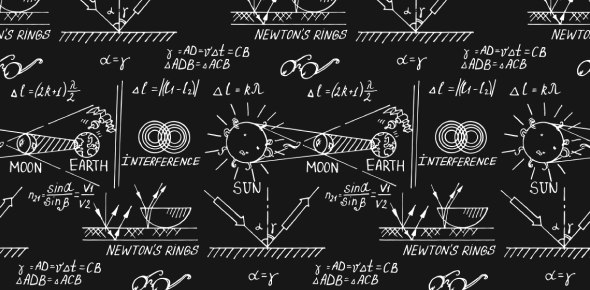# Physics 1320 Mount Exam 2 Practice Problems

41 Questions | Attempts: 72Settings• 1.
Light is emitted when
• A.

Atomic nuclei are made to vibrate

• B.

High-frequency sound waves strike matter

• C.

Electromagnetic waves emanate from matter

• D.

Electron clouds of atoms are forced into oscillation

• 2.
The law of reflection holds for
• A.

Both curved and plane mirrors

• B.

Only plane mirrors

• C.

Only curved mirrors

• D.

Neither of these

• 3.
When light reflects from a surface, there is a change in its
• A.

Frequency

• B.

Speed

• C.

Wavelength

• D.

All of these

• E.

None of these

• 4.
A diver shines light up to the surface of a smooth pond at a 10 degree angle to the normal. Some of the light passes into the air above, and the part that reflects back into the water makes an angle to the normal of
• A.

Less than 10 degrees

• B.

10 degrees

• C.

• D.

More than 10 degrees

• 5.
Interference occurs when two (or more) waves meet while traveling along the same medium.
• A.

True

• B.

False

• 6.
Interference can be constructive or destructive.
• A.

True

• B.

False

• 7.
Interference of two waves at a given location results in the formation of a new pattern which has a greater amplitude than either of the two interfering waves.
• A.

True

• B.

False

• 8.
The meeting of a trough of one wave with a trough of another wave results in destructive interference.
• A.

True

• B.

False

• 9.
Young's experiment provided evidence that light exhibits particle-like behavior.
• A.

True

• B.

False

• 10.
The only way for two waves to interfere constructively is for a crest to meet a crest or a trough to meet a trough.
• A.

True

• B.

False

• 11.
Young's experiment depends upon the use of light from two sources.
• A.

True

• B.

False

• 12.
Refraction results from differences in light's
• A.

Frequency

• B.

Speed

• C.

Incident angles

• D.

All of these

• 13.
The average speed of light is greatest in
• A.

Green glass

• B.

Red glass

• C.

Blue glass

• D.

Orange glass

• E.

Is the same in all of these

• 14.
The critical angle is least in which of the following?
• A.

Vacuum (empty space)

• B.

Water

• C.

Glass

• D.

Diamond

• 15.
A type of lens that spreads out parallel light is a
• A.

Converging lens

• B.

Diverging lens

• C.

Combination of converging-diverging lens

• 16.
A person standing waist-deep in a swimming pool appears to have short legs because of the light
• A.

Absorption

• B.

Diffraction

• C.

Reflection

• D.

Refraction

• E.

Interference

• 17.
Different colors are dispersed by a prism because different colors in the prism have different
• A.

Frequencies

• B.

Speeds

• C.

Energies

• D.

Directions

• E.

None of these

• 18.
According to Huygen's principle, every point on a wave
• A.

Is a diffraction source

• B.

Is a superposition of every other part of a wave

• C.

Behaves as a source of new waves

• D.

All of these

• 19.
Diffraction is more pronounced through relatively
• A.

Large openings

• B.

Small openings

• C.

Same for each

• 20.
Waves diffract the least when their wavelengths are
• A.

Long

• B.

Short

• C.

Both diffract the same

• 21.
Diffraction is a result of
• A.

Dispersion

• B.

Reflection

• C.

Refraction

• D.

Polarization

• E.

Interference

• 22.
Polarization is a property of
• A.

Transverse waves

• B.

Longitudinal waves

• C.

Both

• D.

Neither

• 23.
Monochromatic light is light of a single
• A.

Color

• B.

Frequency

• C.

Wavelength

• D.

All of these

• 24.
The spacing between double-slit interference fringes is increased if the slits are
• A.

Narrower

• B.

Closer together

• C.

Wider

• D.

Farther apart

• 25.
If an object is placed inside the focal point of a diverging lens, the image is
• A.

Virtual, upright and reduced

• B.

Real, upright and reduced

• C.

Virtual, inverted and reduced

• D.

Virtual, upright and enlarged

## Related TopicsBack to top
×

Wait!
Here's an interesting quiz for you.